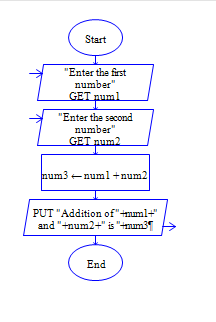# Java Program to Addition of two numbers

Get two integer numbers, add both the integers and display the sum.

Sample Input 1:

5 6

Sample Output 1:

11

Sample Input 2:

65 4

Sample Output 2:

69

#### Flow Chart Design#### Program or Solution

``` import java.util.*; class Addition { public static void main(String args[]) { int Input1,Input2,sum=0; Scanner sc=new Scanner(System.in); System.out.println("Enter The Input 1:"); Input1=sc.nextInt(); System.out.println("Enter The Input 2:"); Input2=sc.nextInt(); sum=Input1+Input2; System.out.println("The Output Is:\n"+sum); } } ```

#### Program Explanation

Get two integers Input1 and Input2 (using scanner class) Add Input1 and Input2, then store the sum (sum=Input1+Input2) print the value of sum (using system.out.println)